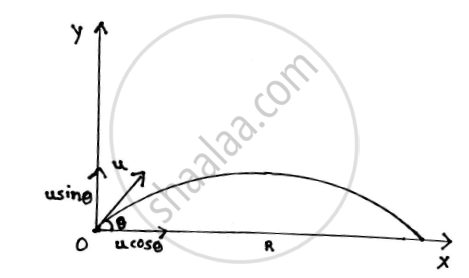Advertisement Remove all ads

# Derive the Equation of Path of a Projectile and Hence Show that Equation of Path of Projectile is a Parabolic Curve. - Engineering Mechanics

Derive the equation of path of a projectile and hence show that equation of path of projectile is a parabolic curve.

Advertisement Remove all ads

#### SolutionLet us assume that a projectile is fired with an initial velocity u at an angle θ with the horizontal. Let t be the time of flight. Let x be the horizontal displacement and y be the vertical displacement.

HORIZONTAL MOTION :

In the horizontal direction,the projectile moves with a constant velocity.

Horizontal component of initial velocity u is u.cosθ

Displacement = velocity x time

x = u.cosθ x t

t=x/(ucosθ)

VERTICAL MOTION OF PROJECTILE:

In the vertical motion,the projectile moves under gravity and hence this is an accelerated motion.

Vertical component of initial velocity u = u.sinθ Using kinematics equation :

s= u_yt+1/2 x  a  x  t^2

y=usinθ xx x/(ucosθ)-1/2xx g xx (x/(uosθ))^2

y=xtanθ-(gx^2)/(2u^2 cos^2θ)

This is the equation of the projectile

This equation is also the equation of a parabola

Thus, proved that path traced by a projectile is a parabolic curve.

Concept: Velocity and Acceleration in Terms of Rectangular Co-ordinate System
Is there an error in this question or solution?
Advertisement Remove all ads

#### APPEARS IN

Advertisement Remove all ads
Advertisement Remove all ads
Share
Notifications

View all notifications

Forgot password?